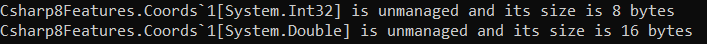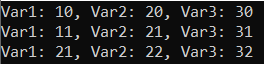# Unmanaged Constructed Types in C#

## Unmanaged Constructed Types in C# 8 with Examples

In this article, I am going to discuss Unmanaged Constructed Types in C# 8 with Examples. Please read our previous article where we discussed Null-Coalescing Assignment Operator in C# 8 with Examples. In C# 7.3 and earlier, a constructed type (a type that includes at least one type of argument) can’t be an unmanaged type. Starting with C# 8.0, a constructed value type is unmanaged if it contains fields of unmanaged types only.

##### What is Unmanaged Type in C#?

A type is called unmanaged type when it is being used in an unsafe context. This is true for many built-in basic types. A type is an unmanaged type, if it is any of the following types:

1. sbyte, byte, short, ushort, int, uint, long, ulong, char, float, double, decimal, or bool
2. Any enum type
3. Any pointer types
4. Any user-defined struct type that contains fields of unmanaged types only.
##### What is Constructed Type in C#?

A type is called constructed type if it is generic and the type parameter is already defined. For example, List<string> is a constructed type while List<T> is not. This is because the string is already defined as a data type whereas T is not already defined as a data type. In C# 7.3 and earlier, a Constructed Type can’t be an Unmanaged Type. Starting with C# 8.0, a constructed value type is unmanaged if it contains fields of unmanaged types only.

Beginning with C# 7.3, you can use the unmanaged constraint to specify that a type parameter is a non-pointer, non-nullable unmanaged type. Beginning with C# 8.0, a constructed struct type that contains fields of unmanaged types only is also unmanaged, as the following example shows:

```using System;
namespace Csharp8Features
{
public struct Coords<T>
{
public T X;
public T Y;
}

public class UnmanagedTypes
{
public static void Main()
{
DisplaySize<Coords<int>>();
DisplaySize<Coords<double>>();
}

private unsafe static void DisplaySize<T>() where T : unmanaged
{
Console.WriteLine(\$"{typeof(T)} is unmanaged and its size is {sizeof(T)} bytes");
}
}
}
```
###### Output:A generic struct may be the source of both unmanaged and managed constructed types. The preceding example defines a generic struct Coords<T> and presents examples of unmanaged constructed types. An example of a managed type is Coords<object>. It’s managed because it has the fields of the object type, which is managed. If you want all constructed types to be unmanaged types, use the unmanaged constraint in the definition of a generic struct as shown below.

```public struct Coords<T> where T : unmanaged
{
public T X;
public T Y;
}```
##### Unmanaged Constructed Types:

Let’s consider the following example of an unmanaged constructed type that it was not possible to declare before C# 8.0.

```public struct Foo<T>
{
public T var1;
public T var2;
public T var3;
}```

The above example defines the generic Foo<T> type. The Foo<double> type is an unmanaged type in C# 8.0, and for any unmanaged type, you can create a pointer to a variable of this type or allocate a block of memory on the stack for instances of this type as shown below.

```Span<Foo<int>> bars = stackalloc[]
{
new Foo<int> { var1 = 10, var2 = 20, var3 = 30 },
new Foo<int> { var1 = 11, var2 = 21, var3 = 31 },
new Foo<int> { var1 = 21, var2 = 22, var3 = 32 },
};
```
##### Complete Example Code:
```using System;
namespace Csharp8Features
{
public struct Foo<T>
{
public T var1;
public T var2;
public T var3;
}

public class UnmanagedTypes
{
public static void Main()
{
// Block of memory
Span<Foo<int>> bars = stackalloc[]
{
new Foo<int> { var1 = 10, var2 = 20, var3 = 30 },
new Foo<int> { var1 = 11, var2 = 21, var3 = 31 },
new Foo<int> { var1 = 21, var2 = 22, var3 = 32 },
};

foreach (var bar in bars)
{
Console.WriteLine(\$"Var1: {bar.var1}, Var2: {bar.var2}, Var3: {bar.var3}");
}
}
}
}
```
###### Output:Note: A generic struct may be the source of both unmanaged and not unmanaged constructed types. The above example defines a generic struct Foo<T> and presents an unmanaged constructed type.

##### Points to Remember:
1. This feature is a performance enhancement.
2. Constructed value types are now unmanaged if it only contains fields of unmanaged types.
3. This feature means that you can do things like allocate instances on the stack

In the next article, I am going to discuss Stackalloc in Nested Expressions in C# 8 with Examples. Here, in this article, I try to explain Unmanaged Constructed Types in C# 8 with Examples. I hope you enjoy this article.